## The outer layer of cable on a cable reel is 16.2 cm from the center of the reel. The reel is initially stationary and can rotate about a fix

Question

The outer layer of cable on a cable reel is 16.2 cm from the center of the reel. The reel is initially stationary and can rotate about a fixed axis. It takes 3.6 seconds to pull off 3.7 meters of cable. Assume that the cable is pulled with a constant force (constant acceleration) and that the change in the effective radius is negligible.

How many revolutions did the reel make in 3.6 seconds? (no units)

However, I am really having trouble figuring out parts B and C to this problem

B) What is the angular speed of the reel after 3.6 seconds?

C)What is the angular acceleration of the reel?

in progress 0
2 months 2021-08-06T05:13:45+00:00 2 Answers 0 views 0

Explanation:

B)

We can solve this problem by taking into account that (as in the uniformly accelerated motion)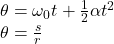( 1 )

where w0 is the initial angular speed, α is the angular acceleration, s is the arc length and r is the radius.

In this case s=3.7m, r=16.2cm=0.162m, t=3.6s and w0=0. Hence, by using the equations (1) we have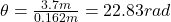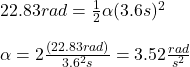to calculate the angular speed w we can use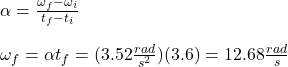C) We can use our result in B)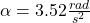I hope this is useful for you

regards

b)the angular speed after 3.6s is is 12.7rad/s

c) the angular acceleration of the reel is 3.52rad/s²

Explanation:

b)

The angle in radian is represented as follows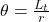r = 16.2cm = 0.162m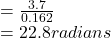now,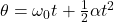where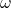is the angular speed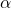is the angular acceleration

t is the time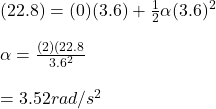the first equation of motion is represeented by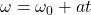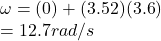the angular speed after 3.6s is is 12.7rad/s

c)

from the above calculation , angular acceleration is calculated as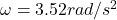Therefore, the angular acceleration of the reel is 3.52rad/s²